# Creating Bayesian Networks using BNS

BNS is a library of classes written to create Bayesian Networks, as described in the book “Probabilistic Networks and Expert Systems,” by R.  G. Cowell, A. P. Dawid, S. L. Lauritzen, and D. J. Spiegelhalter.  As of this writing, there are two versions of BNS, one written as C++ templates, and another in the Java language.

The documentation for the C++ version of the code is in http://jmvidal.ece.sc.edu/targetshare/bayes/cpp/

The documentation for the Java version of the code is in: http://jmvidal.ece.sc.edu/targetshare/bayes/java/

The classes are:

• CBNSNode

• CBNSCliqueBase
• CBNSClique
• CBNSSeparator
• CBNSCharge

• CBNSProbVector
• CBNSProbTable

• CBNSGraph
• CBNSJunctionTree

• CBNSNetwork

This document describes how to use the classes from the C++ implementation.

Creating a Bayesian Network from the C++ Classes:

1. Create a CBNSNetwork object
1. This is done by object instantiation

·        CBNSNetwork nw;

1. Create nodes
1. This is done by using the CBNSNode Class

·        CBNSNode *nodeA = new CBNSNode(“node_name”, nNoOfStates);

·        node_name is of (STL) string type, and nNoOfSates is an integer

·        It is assumed that the states are discrete and are of STL string type. Further implementation would involve some minor modifications.

1. Add the nodes to the network
1. This is done by using the AddNode function in CBNSNetwork Class

·        nodeA is a pointer to a CBNSNode Object

·        nodeA and nodeB are CBNSNode pointers and the link is from the nodeA to the nodeB, ie., (nodeA ® nodeB)

·        It is assumed that the link is directed.

·        The link is added to network, parent node and child node

·        Adding the Link automatically changes the size of the probability tables of the nodes.

1. The next step would be to create the probability tables for all the nodes. The steps involved in creating the probability tables for the node are shown below
1. Create probability vectors equal to the number of states of the node

·        CBNSProbVector vNodeA1, vNodeA2;

1. Add the probability values to the vector

·        VNodeA1.push_back(0.1); vNodeA1.push_back(0.2);

·        VnodeA2.push_back(0.5); vNodeA2.push_back(0.3);

1. Create an object of vector<CBNSProbVector*>

·        vector<CBNSProbVector*> vA;

1. Add the CBNSProbVectors to this.

·        vA.push_back(&vNodeA1); vA.push_back(&vNodeA2);

1. Set the Contingency table / Probability table of the node using this

·        nodeA.SetContingencyTable(vA);

·        the above function returns true if the probability table is set successfully

1. The probability table can be set using variable list arguments, explained later.

1. Once the probability tables for all the nodes, the network is ready for compilation
1. Compile the network

·        nw.Compile( );

·        This function creates the Junction Tree using minimum degree algorithm, Creates potentials for the cliques and makes the tree sum consistent.

·        To print the node probabilities, use nw.PrintNodeProbabilities( ).

1. The evidence for any node can be set as follows
1. nw.SetEvidence(nodeA, 1);
2. where the integer 1 is the second state of the node
3. When the evidence is set, the probability of that state is set to one and the probability of the remaining states is set to zero.
2. Once the Evidence is set, the network needs propagation for attaining sum consistency
1. Use nw.Propagate( );
2. Print the node probabilities after propagation for comparison.

# Common Errors

1. Make sure to delete the objects created, for eg. nodes, links and tables.
2. The second possible type of error could be associated to the probability tables for nodes. This could go wrong in number of ways.
1. When using a variable argument list, make sure that the number of entries are correct in the function argument list.
2. When using the vector of CBNSProbVectors, the number of CBNSProbVector objects should be same as the number of states of the node and the size of each vector should be equal to the product of the number of states of the parents.

i.      For eg., say nodeA has two parents, nodeB and nodeC. NodeB has 4 states, nodeC has 5 states, and nodeA has 3 states, then

.            The probability vector size should be 20 (4 x 5)

.            Three such probability vectors corresponding to each probability state of nodeA should be added to vector< CBNSProbVector* >

#### Design of Probabilty Tables

Suppose the NodeA has 3 states, NodeB has 3 states, and NodeC has 2 States. Let the graph be as shown in the figure below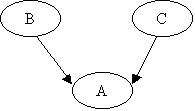NodeA Probability Table:

A ¯

B: State 0

B: State1

B: State 2

C:State 0

C:State 1

C:State 0

C:State 1

C:State 0

C:State 1

##### State 0

B:0, C:0

B:0, C:1

B:1, C:0

B:1, C:1

B:2, C:0

B:2, C:1

State 1

B:0, C:0

B:0, C:0

B:1, C:0

B:1, C:0

B:2, C:0

B:2, C:1

State 2

B:0, C:0

B:0, C:0

B:1, C:0

B:1, C:0

B:2, C:0

B:2, C:1

###### The data in the table is shown below
 CBNSProbVector vA0 B:0, C:0 B:0, C:1 B:1, C:0 B:1, C:1 B:2, C:0 B:2, C:1 CBNSProbVector vA1 B:0, C:0 B:0, C:0 B:1, C:0 B:1, C:0 B:2, C:0 B:2, C:1 CBNSProbVector vA2 B:0, C:0 B:0, C:0 B:1, C:0 B:1, C:0 B:2, C:0 B:2, C:1

The probability table of NodeA is stored in the vector of pointers to CBNSProbVector.

###### The pictorial representation of the table is shown below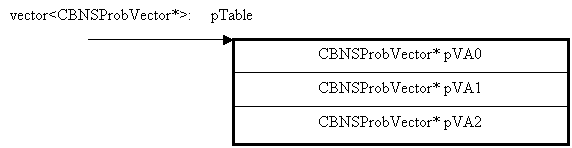CBNSProbVector* pVA0 represents a vector containing the conditional probability values of the zeroth state of NodeA. Similarly pVA1 represents conditional probability values of first state, and so on.

Conditional probablity values of zeroth state of NodeA is represented below,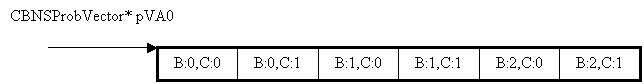The Conditional probability values of Node A are given as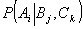, which is read as the probability of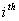state of NodeA, givenstate of B, andstate of C.

For the scenario under discussion, i = 0,…2, j = 0,…2, and k = 0,…,1

Example (From Finn Jensen: An introduction to Bayesian Networks)

Holmes leaves his house. He observes that his grass is wet (H). Is it due to rain (R)? or has he forgotten to turn the Sprinkler (S) off? Later on, as a second observation, Holmes notes that Mr. Watson grass (W) is also wet. Holmes is almost certain that it rained.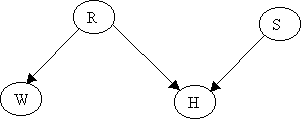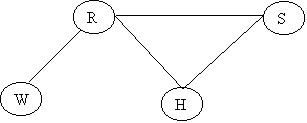The Junction Tree will be: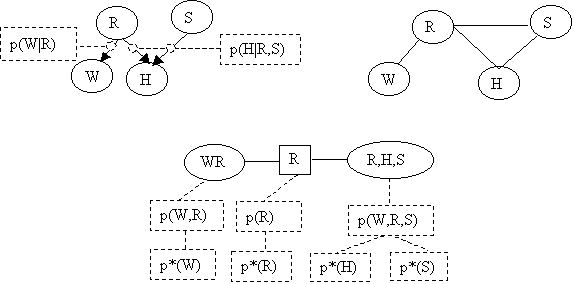Assume the following priors:

p(R) = (0.2, 0.8) [ y, n ]

p(S) = (0.1, 0.9) [ y, n ]

The conditionals are:

 R=y R=n R=y R=n W=y 1.0 0.2 S=y (1, 0) (0.9, 0.1) W=n 0.0 0.8 S=n (1, 0) (0,    1.0)

P(W|R)                                                P(H|R,S)

The vectors in the table represent (H=y, H=n).

Converting  P(H|R,S)  into the format we have used:

 R=y R=n S=y S=n S=y S=n H=y 1.0 1.0 0.9 0.0 H=n 0.0 0.0 0.1 1.0

From the graph we can obtain the joint probability for the CLQ(H,R,S)

P(H,R,S) = P(H|R,S)P(R,S)

Since R and S are independent (H is a collider)

P(H,R,S) = P(H|R,S)P(R)P(S)

We can obtain the prior probability table for p(H,R,S) using the expression

p(H,R,S) = p(H|R,S)p(R)p(S)

e.g.,

p(H=y,R=y,S=n)          = p(H=y|R=y,S=n)*p(R=y)*p(S=n)

= 1.0*0.2*0.9 = 0.18

p(H=n,R=n,S=n)          = 1.0*0.8*0.9 = 0.72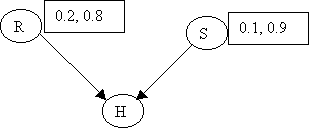Summarizing in table form:

 R=y R=n S=y S=n S=y S=n H=y 0.02 0.18 0.072 0.0 H=n 0.0 0.0 0.008 0.72

We can obtain p(H) by marginalizing R and S out of P(H,R,S)

 R=y R=n S=y S=n S=y S=n p(H) H=y 0.02 0.18 0.072 0.0 0.272 H=n 0.0 0.0 0.008 0.72 0.728

P(H) = (0.272, 0.728)

Doing the same for p(W), starting off with p(W,R)

p(W,R) = p(W|R)p(R)

p(W,R) = p(w=y|r=y)p(R=y)=1.0*0.2 = 0.20

p(W,R) = p(w=y|r=n)p(R=y)=0.2*0.8 = 0.16

p(W,R) = p(w=n|r=y)p(R=n)=0.0

p(W,R) = p(w=n|r=n)p(R=n)=0.8*0.8 = 0.64

 R=y R=n p(W) W=y 0.2 0.16 0.36 W=n 0.0 0.64 0.64

This completes the initialization phase. The network looks like: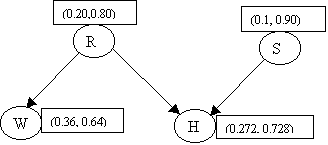Suppose we observe that H=y. A new table p*(H,R,S) is needed.

This table is created by zeroing the entries for H=n from the table p(H,R,S), and then dividing by p(H=y)

 R=y R=n S=y S=n S=y S=n H=y 0.02*1/.272 0.18*1/.272 0.072*1/.272 0.0*1/.272 H=n 0.0*0.0 0.0*0.0 0.008*0 0.0*0

 R=y R=n S=y S=n S=y S=n H=y 0.074 0.662 0.264 0.00 H=n 0.00 0.00 0.00 0.00

We marginalize from p*(H,R,S) on (H,S) and on (H,R) to obtain

p*(R)= (0.736, 0.264) and  p*(S)=(0.339, 0.662).

Now we use the new p*(R) to update p(W,R), using

p*(W,R) = p(W|R)*p*(R) = p(W,R)[ p*(R)/p(R) ]

 R=y R=n W=y 0.2*0.736/0.2 0.16*0.264/0.8 W=n 0.0 0.64*0.264/0.8

 R=y R=n W=y 0.736 0.0528 W=n 0.0 0.2112

The code below implements the concepts described above:

void Testing_HolmesWatsonExample()

{

cout << "Simple CBNSNetwork Test 1. Holmes and Watson Example:\n";

CBNSNode nodeR("R", 2);

CBNSNode nodeS("S", 2);

CBNSNode nodeH("H", 2);

CBNSNode nodeW("W", 2);

CBNSNetwork nw;

vector<double> vR1, vR2, vS1, vS2, vH1, vH2, vW1, vW2;

vR1.push_back(0.2);vR2.push_back(0.8);

vS1.push_back(0.1);vS2.push_back(0.9);

vH1.push_back(1.0);vH2.push_back(0.0);

vH1.push_back(1.0);vH2.push_back(0.0);

vH1.push_back(0.9);vH2.push_back(0.1);

vH1.push_back(0.0);vH2.push_back(1.0);

vW1.push_back(1.0);vW2.push_back(0.0);

vW1.push_back(0.2);vW2.push_back(0.8);

CBNSProbVector pv_R1(vR1), pv_R2(vR2), pv_S1(vS1),

pv_S2(vS2), pv_H1(vH1), pv_H2(vH2),pv_W1(vW1), pv_W2(vW2);

vector<CBNSProbVector*> vR, vS, vH, vW;

vR.push_back(&pv_R1); vR.push_back(&pv_R2);

vS.push_back(&pv_S1); vS.push_back(&pv_S2);

vH.push_back(&pv_H1); vH.push_back(&pv_H2);

vW.push_back(&pv_W1); vW.push_back(&pv_W2);

cout << "Validating the Probability tables: "

<< nodeR.SetContingencyTable(vR) << nodeS.SetContingencyTable(vS);

cout << nodeH.SetContingencyTable(vH) << nodeW.SetContingencyTable(vW)

<< endl;

nw.Compile();

nw.PrintNodeProbabilities();

cout << "\n\n\n";

}

Example: Visit to Asia

Tuberculosis and lung cancer can cause dyspnea with equal likelihood.  The same is true for a positive Xray (a positive Xray is also equally likely given either tuberculosis or lung cancer). Bronchitis is another cause of dyspnea.  A recent visit to Asia increases the likelihood of tuberculosis, while smoking is a possible cause for both cancer and bronchitis. The pictorial representation of the network is: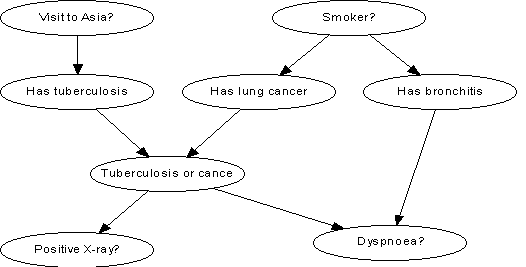## The code that implements the Visit to Asia Example

int main(int argc, char* argv[])

{

double start_time = clock();

Testing_Visit_To_Asia_Example();

cout << "***** Testing for memory leak in the program *****\n\n";

cout << "CBNSCharge::m_nCounter = "<< CBNSCharge::m_nCounter << endl;

cout << "CBNSClique::m_nCounter  = " << CBNSClique::m_nCounter<< endl;

cout << "CBNSCliqueBase::m_nCounter = " << CBNSCliqueBase::m_nCounter<< endl;

cout << "CBNSGraph::m_nCounter        = "<< CBNSGraph::m_nCounter<< endl;

cout << "CBNSJunctionTree::m_nCounter = "<< CBNSJunctionTree::m_nCounter << endl;

cout << "CBNSNetwork::m_nCounter      = "<< CBNSNetwork::m_nCounter << endl;

cout << "CBNSProbTable::m_nCounter    = "<< CBNSProbTable::m_nCounter << endl;

cout << "CBNSProbVector::m_nCounter   = "<< CBNSProbVector::m_nCounter << endl;

cout << "CBNSSeparator::m_nCounter    = " << CBNSSeparator::m_nCounter << endl;

cout << "CBNSNode::m_nCounter         = "<< CBNSNode::m_nCounter << endl;

// The time is written to cerr so the time can be seen when the output

// is directed to a file.

cerr << "\nTotal Time: " << (double(clock()) - start_time) / 1000.0 << " seconds.\n\n";

return 0;

}

## The function to test the Visit to Asia Example

void Testing_Visit_To_Asia_Example()

{

cout << "***** Testing Visit to Asia Example *****\n\n";

//Creating Nodes with  States

CBNSNode nodeA("A", 2);

CBNSNode nodeB("B", 2);

CBNSNode nodeC("C", 2);

CBNSNode nodeD("D", 2);

CBNSNode nodeE("E", 2);

CBNSNode nodeF("F", 2);

CBNSNode nodeG("G", 2);

CBNSNode nodeH("H", 2);

CBNSNetwork nw;

//Creating Tables for all the nodes

vector<CBNSProbVector*> PTableA, PTableB, PTableC, PTableD, PTableE, PTableF, PTableG, PTableH;

/*********** Probability Table for Node A *********/

CBNSProbVector vA1, vA2;

vA1.push_back(0.01); vA2.push_back(0.99);

PTableA.push_back(&vA1); PTableA.push_back(&vA2);

/*********** Probability Table for Node B **********/

CBNSProbVector vB1, vB2;

vB1.push_back(0.05); vB2.push_back(0.95);

vB1.push_back(0.01); vB2.push_back(0.99);

PTableB.push_back(&vB1); PTableB.push_back(&vB2);

/*********** Probability Table for Node C **********/

CBNSProbVector vC1, vC2;

vC1.push_back(1.00); vC2.push_back(0.00);

vC1.push_back(1.00); vC2.push_back(0.00);

vC1.push_back(1.00); vC2.push_back(0.00);

vC1.push_back(0.00); vC2.push_back(1.00);

PTableC.push_back(&vC1); PTableC.push_back(&vC2);

//*********** Probability Table for Node D **********/

CBNSProbVector vD1, vD2;

vD1.push_back(0.98); vD2.push_back(0.02);

vD1.push_back(0.05); vD2.push_back(0.95);

PTableD.push_back(&vD1); PTableD.push_back(&vD2);

/*********** Probability Table for Node E **********/

CBNSProbVector vE1, vE2;

vE1.push_back(0.10); vE2.push_back(0.90);

vE1.push_back(0.01); vE2.push_back(0.99);

PTableE.push_back(&vE1); PTableE.push_back(&vE2);

/*********** Probability Table for Node F **********/

CBNSProbVector vF1, vF2;

vF1.push_back(0.5); vF2.push_back(0.5);

PTableF.push_back(&vF1); PTableF.push_back(&vF2);

/*********** Probability Table for Node G **********/

CBNSProbVector vG1, vG2;

vG1.push_back(0.60); vG2.push_back(0.40);

vG1.push_back(0.30); vG2.push_back(0.70);

PTableG.push_back(&vG1); PTableG.push_back(&vG2);

/*********** Probability Table for Node H **********/

CBNSProbVector vH1, vH2;

vH1.push_back(0.90); vH2.push_back(0.10);

vH1.push_back(0.70); vH2.push_back(0.30);

vH1.push_back(0.80); vH2.push_back(0.20);

vH1.push_back(0.10); vH2.push_back(0.90);

PTableH.push_back(&vH1); PTableH.push_back(&vH2);

//*********** Setting the Probability table for all the Nodes ******//

cout << "Validating Node A Table: "<<

((nodeA.SetContingencyTable(PTableA)==0)?"Wrong":"Right") << endl;

cout << "Validating Node B Table: "<< ((nodeB.SetContingencyTable(PTableB)==0)?"Wrong":"Right") << endl;

cout << "Validating Node C Table: "<< ((nodeC.SetContingencyTable(PTableC)==0)?"Wrong":"Right") << endl;

cout << "Validating Node D Table: "<< ((nodeD.SetContingencyTable(PTableD)==0)?"Wrong":"Right") << endl;

cout << "Validating Node E Table: "<< ((nodeE.SetContingencyTable(PTableE)==0)?"Wrong":"Right") << endl;

cout << "Validating Node F Table: "<< ((nodeF.SetContingencyTable(PTableF)==0)?"Wrong":"Right") << endl;

cout << "Validating Node G Table: "<< ((nodeG.SetContingencyTable(PTableG)==0)?"Wrong":"Right") << endl;

cout << "Validating Node H Table: "<< ((nodeH.SetContingencyTable(PTableH)==0)?"Wrong":"Right") << endl;

cout << "\n\n";

//Compiling the network

nw.Compile();

cout << "\n**** Printing Node Probabilities *****\n";

//Printing the node probabilities

nw.PrintNodeProbabilities();

//Setting the Evidence and propagating

cout << "\n***** Setting Evidence of Node A to State 0 *****\n";

nw.SetEvidence(&nodeA, 0);

nw.Propagate();

//Printing the node probabilities after propagation

nw.PrintNodeProbabilities();

cout << "\n\n\n";

}

### Results

***** Testing Visit to Asia Example *****

Validating Node A Table: Right

Validating Node B Table: Right

Validating Node C Table: Right

Validating Node D Table: Right

Validating Node E Table: Right

Validating Node F Table: Right

Validating Node G Table: Right

Validating Node H Table: Right

Root Clique: {A, B}

**** Printing Node Probabilities *****

A                : [ 0.01 0.99 ]

B                : [ 0.0104 0.99 ]

C                : [ 0.0648 0.935 ]

D                : [ 0.11 0.89 ]

E                : [ 0.055 0.945 ]

F                : [ 0.5 0.5 ]

G                : [ 0.45 0.55 ]

H                : [ 0.436 0.564 ]

***** Setting Evidence of Node A to State 0 *****

A                : [ 1 0 ]

B                : [ 0.05 0.95 ]

C                : [ 0.102 0.898 ]

D                : [ 0.145 0.855 ]

E                : [ 0.055 0.945 ]

F                : [ 0.5 0.5 ]

G                : [ 0.45 0.55 ]

H                : [ 0.45 0.55 ]

***** Testing for memory leak in the program *****

CBNSCharge::m_nCounter                  = 0

CBNSClique::m_nCounter       = 0

CBNSCliqueBase::m_nCounter            = 0

CBNSGraph::m_nCounter                    = 0

CBNSJunctionTree::m_nCounter          = 0

CBNSNetwork::m_nCounter                = 0

CBNSProbTable::m_nCounter             = 0

CBNSProbVector::m_nCounter           = 0

CBNSSeparator::m_nCounter              = 0

CBNSNode::m_nCounter                     = 0